JOINProblem Statement
Contest: Marathon Match 1
Problem: VehicleRecognition

Problem Statement

The purpose of this challenge is to write a function which automatically classifies aerial images based on whether they contain a vehicle.

A set of approximately 1000 JPEG images of vehicles and 3000 JPEG images of background are provided for developing the algorithm. Each image is in the standard sRGB color space. You can download the set here. The images in "vehicles" folder contain vehicles and the images in "background" folder contain only background, but no vehicles. The files with extension ".jpg" are usual JPEG images which you can view using virtually any image viewer software.

For convenience, you will also be provided with an utility which can be used to transform ".jpg" files to ".raw" format. This is not a widely known format, but rather a custom format designed specifically for this problem to simplify the process of inputting the image data. Each ".raw" file contains several whitespace-separated integers. The first two integers are W and H -- the width and the height of the image (in pixels). The description of pixels in column-major order follows, i.e., first, the pixels of column 0 (the leftmost column) are described from top to bottom, then the pixels of column 1, column 2, and so on. Each pixel is given by 3 integers from 0 to 255, inclusive. These integers are the red, the green and the blue component of the pixel, in this exact order. You can download the utility here.

Your class will need to implement the method classifyImage that takes a int[] image as its input. This int[] contains a description of an image. The int[] will contain the same integers and in the same order as a ".raw" file for this image. In other words, the first two integers are the width and the height of the image, and the next integers describe the pixels of the image in column-major order, 3 integers per pixel -- the red, the green and the blue component. You will need to return a double value between 0.0 and 1.0, inclusive, that indicates the level of your insurance that the image contains a vehicle, where 0.0 means that you are absolutely sure that there is no vehicle and 1.0 means you are absolutely sure that there is a vehicle. Please read the section about scoring to get a better understanding of what the return value means.

There will be 10 examples test cases and approximately 400 submission test cases. Both these sets will contain only the images chosen among the ones provided to you. Therefore the submission score does not necessarily reflect how good is your algorithm, since it could be that you overtuned the algorithm to the given images. Once the coding is over, all submissions will be run against approximately 400 system test cases. None of the system test case images is provided to you in advance, but you can assume a certain level of similarity between the images in system tests and the images provided to you.

Scoring

The score for a single test case will be calculated as follows. Let R be the the value returned by your classifyImage method. If the image in this test case does not contain a vehicle, the score will be equal to R, otherwise, if it contains a vehicle, the score will be equal to 100.0 + R. For example, a score of 100.7 means that your method returned 0.7 and this image contained a vehicle and a score of 0.3 means that your method returned 0.3 and this image did not contain a vehicle.

If your solution was not able to produce the answer for a test case (due to exceeding time limit, exceeding memory limit, crash, etc.), it will be assumed that it returned 0.0. Also, if the return value is greater than 1.0, it will be assumed that your solution returned 1.0, and the return values less than 0.0 will be considered the same as 0.0.

The overall score is calculated as follows. Let ret(I) be the return of your classifyImage method for the image I. Consider V - the set of all test cases where the corresponding image contains a vehicle, and B - the set of all test cases where the image contains background only. The scoring algorithm will check all pairs of images (I1, I2), where I1 is from B and I2 is from V. It will use an internal variable S initially set to 0. Each pair where ret(I1) < ret(I2) makes S increased by 1.0, each pair where ret(I1) = ret(I2) increases S by 0.5 and pairs where ret(I1) > ret(I2) do not contribute anything to S. Your final score will be equal to S / P, where P is the total number of all pairs (I1, I2), i.e., P = (number of images in V) * (number of images in B).

Note that calculations in double type are subject to rounding errors, therefore our implementation of the scoring algorithm will consider two double values equal if the difference between them is less than or equal to 1e-9. For the reference, here is the pseudocode:

S = 0
for each image I1 from B
for each image I2 from V
if |ret(I1) - ret(I2)| <= 1e-9
S = S + 0.5
else if ret(I1) < ret(I2)
S = S + 1.0
score = 1000.0 * S / (sizeOf(B) * sizeOf(V))

Note that the overall score ranges from 0.0 to 1000.0, with values close to 500.0 representing pure random guess, and 1000.0 representing a perfect algorithm. Another way to describe the same score is calculating the area under the Receiver Operating Characteristic (ROC) curve and then multiplying it by 1000.0. You can check the following Wikipedia article for details.

Prizes and Conditions

In order to receive the prize money, you will need to fully document the derivation of all parameters internal to your algorithm. If these parameters were obtained from the training data set, you will also need to provide the program used to generate these training parameters. There is no restriction on the programming language used to generate these training parameters. Note that all this data should not be submitted anywhere during the coding phase. Instead, if you win a prize, a TopCoder representative will contact you directly in order to collect this data.

Definition

 Class: VehicleRecognition Method: classifyImage Parameters: int[] Returns: double Method signature: double classifyImage(int[] image) (be sure your method is public)

Notes

-The time limit is 1 second per single test case, the memory limit is 1024 MB. There is no explicit code size limit.
-A separate instance of your class will be created for each test case.

Constraints

-image will contain a description of an image in the format described in the problem statement.
-The height and the width of the image will each be between 50 and 150 pixels, inclusive.

Examples

0)

 Image without a vehicle.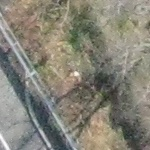1)

 Image containing a vehicle.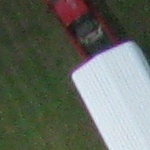2)

 Image without a vehicle.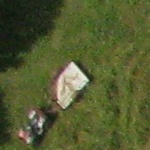3)

 Image containing a vehicle.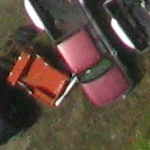4)

 Image without a vehicle.5)

 Image containing a vehicle.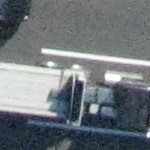6)

 Image without a vehicle.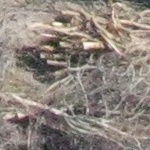7)

 Image containing a vehicle.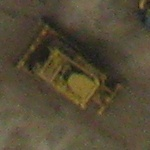8)

 Image without a vehicle.9)

 Image containing a vehicle.This problem statement is the exclusive and proprietary property of TopCoder, Inc. Any unauthorized use or reproduction of this information without the prior written consent of TopCoder, Inc. is strictly prohibited. (c)2010, TopCoder, Inc. All rights reserved.